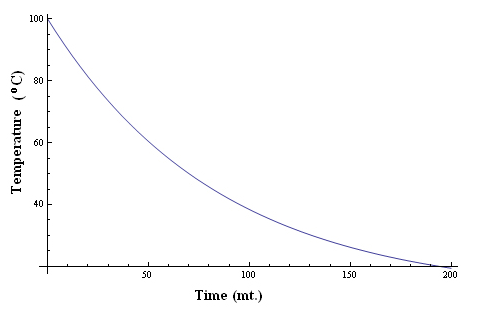According to Newton’s Law of Cooling, the rate of cooling of a body is directly proportional to the difference in temperatures of the body (T) and the surrounding (T0), provided the difference in temperature should not exceed by 300C.

From the above statement,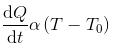For a body of mass m, specific heat s, and temperature T kept in surrounding of temperature T0;Now, the rate of cooling,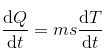Hence,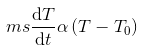Since the mass and the specific heat of the body is taken as constants, the rate of change of temperature with time can be written as,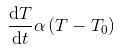The above equation explains that, as the time increases, the difference in temperatures of the body and surroundings decreases and hence, the rate of fall of temperature also decreases.

It can be graphically represented as,